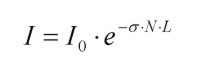# Laser absorption spectroscopy

Light transmitted through some medium (solid, liquid or gaseous) experiences attenuation due to scattering and absorption; the latter being the dominant loss contribution in many cases. Loss measurements can thus be used to deduct the concentration of a gas of interest with known absorption strength in a gas mixture. If local, in-situ values are desired, the gas sample is sucked into a gas cell and measured there. However, if a satellite measures the sunlight reflected from the earth, this also constitutes absorption spectroscopy, with the atmosphere between the earth and satellite being the gas sampled (remote sensing). The light attenuation for sufficiently small absorption is described by the Beer-Lambert-Law:where I is the intensity measured by the detector, I0 is the original intensity entering the gas cell, σ is the absorption cross section of the absorber (in cm² / molec.) and N is the absorber's number density (in molec. / cm³). Those last two multiplied give the absorption coefficient α (α = σ ∙ N, in 1 / cm) and L is the path length of the light-matter interaction (also sometimes called “absorption path length”).